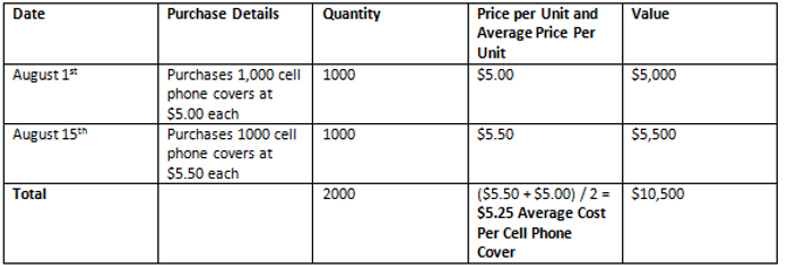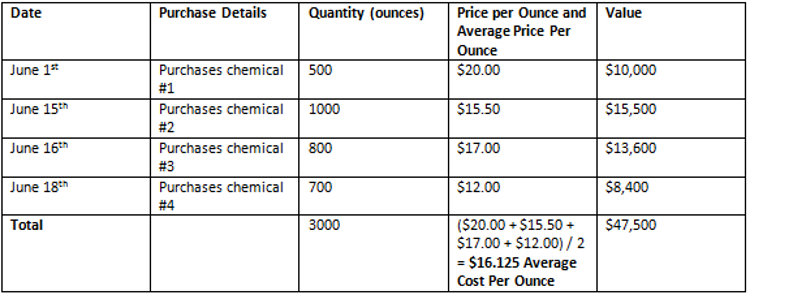Home » Accounting » Average Cost Method of Inventory Examples

# Average Cost Method of Inventory Examples

The average cost method of inventory is used to determine the value of a group of assets or inventory based on the average cost of the assets. It is one of three methods that can be used to value a company’s inventory; the other methods are the FIFO and LIFO methods.

### Average Cost Method of Inventory Video Tutorial With Examples

Each company normally takes a physical inventory count at the end of each month, quarter or year. There also needs to be a way to calculate the value of the inventory.

The weighted average cost of method of inventory is commonly used by manufacturing companies that combine inventories together that cannot be individually identified such as chemicals.

Average Cost Method of Inventory Video Example

Average Cost Method of Inventory Written Example 1
In a simple example below, company ABC Corp. purchases cell phone covers on the following dates:On August 31, ABC Corp. has sold 1200 cell phone covers at \$10.00 each for the month. Therefore the company has 800 cell phone covers remaining.

To determine the value of the remaining inventory using the average cost method of inventory, the \$5.25 average calculated above would be multiplied by 800 (the number of remaining cell phone covers). The result is 800 x \$5.25 = \$4,200. There is \$4,200 worth of inventory left based on this calculation.

Average Cost Method of Inventory Written Example 2
In a slightly more complicated example, XYZ Corp. has four different chemical products that need to have an inventory value assigned to all of them at the end of each month.On June 30th the company has used varying amounts of each chemical to produce a fertilizer product, and there is approximately 1500 ounces remaining from the total of 3000 ounces that were purchased, and the chemicals have been mixed with each other so there is no way to know how many ounces of one specific chemical remain.

Using the average cost method of inventory it is still easy to calculate the value of the remaining inventory. We know that the chemicals have an average cost per ounce of \$16.125. With this number we can make an accurate guess as to the value of the remaining chemicals. With 1500 ounces remaining, this amount is multiplied by the average cost per ounce of \$16.125 or 1500 x \$16.126 = \$24,187.50.

There is approximately \$24,187.50 of inventory left based on this estimate, and although the company does not know the exact amounts of each chemical it has left, it has a good estimate as to the value of the remaining chemical lot.

This type of scenario is where the average cost method of inventory really becomes useful and as previously mentioned, manufacturing companies commonly use it to determine their inventory values after their supplies are mixed and cannot be quantified individually.